## Info

Publication number
JP3595493B2
JP3595493B2 JP2000207849A JP2000207849A JP3595493B2 JP 3595493 B2 JP3595493 B2 JP 3595493B2 JP 2000207849 A JP2000207849 A JP 2000207849A JP 2000207849 A JP2000207849 A JP 2000207849A JP 3595493 B2 JP3595493 B2 JP 3595493B2
Authority
JP
Japan
Prior art keywords
signal
user
weight
matrix
signal processing
Prior art date
Legal status (The legal status is an assumption and is not a legal conclusion. Google has not performed a legal analysis and makes no representation as to the accuracy of the status listed.)
Expired - Fee Related
Application number
JP2000207849A
Other languages
Japanese (ja)
Other versions
JP2002026787A (en
Inventor

Original Assignee

Priority date (The priority date is an assumption and is not a legal conclusion. Google has not performed a legal analysis and makes no representation as to the accuracy of the date listed.)
Filing date
Publication date
Application filed by 三菱電機株式会社 filed Critical 三菱電機株式会社
Priority to JP2000207849A priority Critical patent/JP3595493B2/en
Publication of JP2002026787A publication Critical patent/JP2002026787A/en
Application granted granted Critical
Publication of JP3595493B2 publication Critical patent/JP3595493B2/en
Application status is Expired - Fee Related legal-status Critical
Anticipated expiration legal-status Critical

## Images

•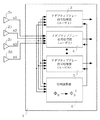••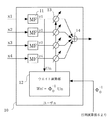•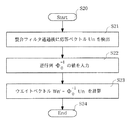•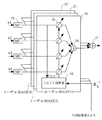•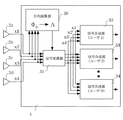•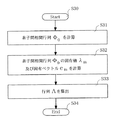•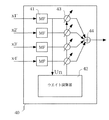•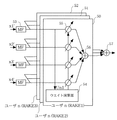•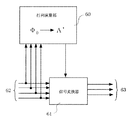•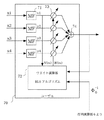•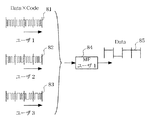•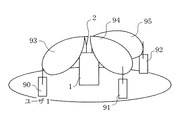•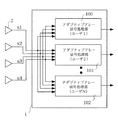•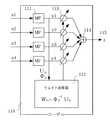•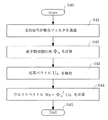•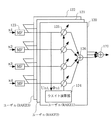## Classifications

• HELECTRICITY
• H04ELECTRIC COMMUNICATION TECHNIQUE
• H04BTRANSMISSION
• H04B7/02Diversity systems; Multi-antenna system, i.e. transmission or reception using multiple antennas
• H04B7/04Diversity systems; Multi-antenna system, i.e. transmission or reception using multiple antennas using two or more spaced independent antennas
• H04B7/08Diversity systems; Multi-antenna system, i.e. transmission or reception using multiple antennas using two or more spaced independent antennas at the receiving station
• H04B7/0837Diversity systems; Multi-antenna system, i.e. transmission or reception using multiple antennas using two or more spaced independent antennas at the receiving station using pre-detection combining
• H04B7/0842Weighted combining
• H04B7/0848Joint weighting
• H04B7/0854Joint weighting using error minimizing algorithms, e.g. minimum mean squared error [MMSE], "cross-correlation" or matrix inversion
• HELECTRICITY
• H04ELECTRIC COMMUNICATION TECHNIQUE
• H04BTRANSMISSION
• H04B1/00Details of transmission systems, not covered by a single one of groups H04B3/00 - H04B13/00; Details of transmission systems not characterised by the medium used for transmission
• H04B1/707Spread spectrum techniques using direct sequence modulation
• HELECTRICITY
• H04ELECTRIC COMMUNICATION TECHNIQUE
• H04BTRANSMISSION
• H04B7/02Diversity systems; Multi-antenna system, i.e. transmission or reception using multiple antennas
• H04B7/04Diversity systems; Multi-antenna system, i.e. transmission or reception using multiple antennas using two or more spaced independent antennas
• H04B7/08Diversity systems; Multi-antenna system, i.e. transmission or reception using multiple antennas using two or more spaced independent antennas at the receiving station
• H04B7/0891Space-time diversity
• H04B7/0897Space-time diversity using beamforming per multi-path, e.g. to cope with different directions of arrival [DOA] at different multi-paths

## Description


TECHNICAL FIELD OF THE INVENTION
The present invention relates to a receiving system in a multiplex wireless communication system in which a plurality of terminals communicate with a base station, and more particularly to an adaptive antenna technology for forming individual antenna patterns for a plurality of users.

[Prior art]
In wireless communication, particularly mobile communication, a plurality of users communicate via one base station. The base station secures a channel for communication of each terminal (user) and sets an environment in which communication can be performed simultaneously. In particular, recently, a code division multiple access (CDMA) scheme has attracted attention as such a multiplexing technique.
In the CDMA system, multiplexing is performed by using different spreading codes for a plurality of users. For example, the transmission signal of user 1 is represented as follows.
S (t) = α · c (t) · d (t) · exp (j2πft) (1)
Here, α represents a propagation constant, c (t) represents a spreading code, d (t) represents data, and f represents a carrier frequency. The data changes its signal value every time unit Tb. The spreading code c (t) changes its code value every time Tc, and G = Tb / Tc (≧ 1) is called a spreading gain. This spreading gain G is usually an integer value.

Thus, in the CDMA system, transmission data is multiplied by a spreading code and transmitted. The spreading code varies from user to user. In the receiver, a matched filter (Matched filter: MF) for identifying a spreading code is prepared, and user identification is performed by each matched filter.
The output signal after passing through the matched filter for user 1 in the baseband band is
Sout (t) = α · G · d (t) (2)
Given by Thus, only the data portion of the output signal is detected.

FIG. 12 shows a case where a plurality of users transmit simultaneously in the CDMA system. In the figure, each user 81, 82, 83 has a different spreading code. In the receiver, a matched filter corresponding to each user is prepared. When the matched filter 84 for the user 1 is used, the signal data 85 of the user 1 is detected, and signals from other users cause minute interference.
As described above, in the CDMA system, signals from a plurality of users can be received simultaneously, but the number of users is limited by the number of spreading codes and the amount of interference. However, in recent mobile communications, with the rapid increase in the number of subscribers, it is necessary to accommodate more users, and a receiver configuration using an adaptive array antenna has been considered as a countermeasure.

FIG. 13 is a diagram showing a usage environment of a CDMA system using an adaptive array antenna. In this figure, 1 is a receiver of a receiving station, 2 is a plurality of antennas provided in the receiving station, and 90, 91 and 92 are terminals.
As shown in this figure, the receiver 1 receives signals from the individual terminals 90, 91, and 92 using a plurality of antennas 2, weights the signals, and combines the signals. When this situation is viewed as an antenna pattern, directional beams 93, 94, and 95 are individually formed for terminals 90, 91, and 92, respectively. As described above, by forming the directional beam corresponding to the signal arrival direction, each user can perform signal reception in a good environment without causing mutual interference.

A CDMA receiver using such an adaptive array is described in, for example, the following documents 1 to 3.
1. Tanaka, Higuchi, Sawabashi, Adachi, "Indoor transmission experimental characteristics of DS-CDMA adaptive array antenna diversity", IEICE Technical Report, Radio Communication Systems Technical Committee, RCS98-53, pp. 19-24, 1998-06.
2. Tanaka, Harada, Sawabashi, Adachi, "Outdoor Transmission Experiment of Adaptive Antenna Array Diversity Reception in DS-CDMA", IEICE Technical Report, Radio Communication Systems Research Group, RCS99-10, p. 19-24, 1999-04.
3. Harada, Tanaka, Ihara, Sawabashi, Adachi, "Outdoor Experiment Results of Adaptive Antenna Array Transmit Diversity in W-CDMA Downlink", IEICE Technical Report, Radio Communication Systems Technical Committee, RCS99-157, pp. 115-121, 1999-11.

Many algorithms for adaptive array algorithms have been proposed so far.
4. Ohgane, Ogawa, "Adaptive Array and Mobile Communication (II)", Journal of the Institute of Electronics, Information and Communication Engineers, Vol. 82 No. 1 pp55-61 1999-1
As described in U.S. Pat. No. 5,867,867, SMI (Sample Matrix Inversion) algorithm, RLS (Recursive Least Squares) algorithm, LMS (Least Mean Square) algorithm and the like are often used. Here, the SMI algorithm and the RLS algorithm both calculate the correlation matrix of the input signal, and have a high convergence speed, but a large amount of calculation, and the LMS algorithm has a small amount of calculation but a slow convergence speed. There is a property that. It should be noted that all of the above-described documents 1 to 3 use the LMS algorithm.

Next, a configuration of a CDMA multi-user receiver using such an adaptive array will be described. Here, it is assumed that an SMI algorithm is used. FIG. 14 is a diagram illustrating a schematic configuration of the receiver 1. In the figure, reference numerals 100 to 102 denote adaptive array signal processing units provided for each user (users 1 to N). FIG. 15 is a diagram showing details of the adaptive array signal processing unit 110 for an arbitrary user n. In the figure, 111 is a matched filter for user n, 112 is an adaptive array weight calculation unit, 113 is a weight multiplication unit, and 114 is signal synthesis. The unit 115 represents the output signal z of the user n. FIG. 16 shows a weight calculation procedure in the adaptive array weight calculation unit 112.

As shown in FIG. 14, signals x1, x2, x3, and x4 received by a plurality of antennas 2 are input to adaptive array signal processing units 100 to 102 corresponding to individual users. The adaptive array signal processing units 100 to 102 of each user are completely independently configured, and the signal processing of each user is configured using the same algorithm.
Here, the details of the adaptive array signal processing unit of an arbitrary user n will be described with reference to FIGS. In FIG. 15, the received signals x1, x2, x3, and x4 from the respective antennas are input to the matched filter 111 for user n, and are despread using the spreading code of the user n (step S41 in FIG. 16). . Although not shown, the received signals x1, x2, x3, and x4 are complex baseband signals obtained by performing synchronous detection after frequency conversion of the received signals from the respective antennas. Is an imaginary part. For each of the despread outputs y1, y2, y3, y4 from the matched filter 111, the adaptive array weight calculator 112 calculates the correlation matrix Φn (step S42) and calculates the response vector Un (step S43). The weight vector Wn is calculated based on (Step S44).

The correlation matrix Φn is given by the following calculation formula.
(Equation 1)
Where E {} represents averaging over a number of data samples and * represents conjugate. The matrix elements represent the inter-antenna correlation for the signal from each matched filter 111, and an averaging process is performed on each data sample. Normally, the averaging process is performed within a time period in which the change in the radio propagation environment is small, and when the propagation environment changes, the value of the correlation matrix Φn is updated.
On the other hand, the response vector Un can be obtained by using a reference signal included in the data of the outputs y1, y2, y3, and y4. Specifically, a matched filter corresponding to the reference signal included in the data is prepared, and signals y1, y2, y3, and y4 are input. The response vector Un can be calculated by using the outputs u1, u2, u3, and u4 as vector elements.
(Equation 2)
Using the correlation matrix Φn and the response vector Un calculated in this manner, the adaptive array weight vector Wn becomes
Wn = Φn-1・ Un (5)
It can be expressed as. It is known that the present weight vector is a value that optimizes the signal quality of the combined output, and can be said to be a theoretically optimal weight vector.
In the adaptive array signal processing unit 110, the weight multiplication unit 113 multiplies the respective elements of the weight vector Wn calculated in this way by the signals y 1, y 2, y 3, and y 4, and synthesizes them by the synthesizer 114 to thereby obtain a synthesized output z 115 Can be obtained.

The CDMA system is characterized in that RAKE reception can be used. Therefore, FIG. 17 shows a configuration of an adaptive array signal processing unit corresponding to user n when RAKE reception, which is a feature of CDMA, is used.
In FIG. 17, reference numeral 120 denotes a RAKE receiver constituting section corresponding to a first tap (corresponding to a first arrival path), and 121 and 122 correspond to second and third taps (corresponding to second and third arrival paths), respectively. And a despread output from a corresponding tap of a matched filter 123 provided for each of the plurality of antennas 2 and corresponding to the user n.
Originally, in CDMA, receivable signals are limited to paths within a time Tc with respect to the first arrival path. However, by using such a RAKE receiver, it is possible to configure a receiver corresponding to each delay path for a delay path having a propagation delay of Tc or more, and to receive each delay path independently. It is possible to do. In addition, by synthesizing the reception outputs of the respective delay paths by the synthesizer 127 at the same timing, it is possible to receive the signals while effectively utilizing the delay paths.

The adaptive array signal processing unit in FIG. 17 includes signal processing units 121 and 122 for the delay path in addition to the signal processing unit 120 for the first arrival path. Each signal processing unit 120 to 122 is supplied with a despread output corresponding to the path from a matching filter 123 provided corresponding to each antenna, and receives a first arrival path, a second arrival path, or a third arrival path. As with the adaptive array signal processing unit 110 shown in FIG. 15, the received signal of the incoming path is multiplied by the weight vector calculated by the weight calculation unit 124 in the weight multiplication unit 125 and synthesized by the synthesizer 126. As a result, a combined output of the reception signal of the path is obtained. Then, all the signals synthesized for each path are further synthesized in the synthesizer 127. In the combining by the combiner 127, the maximum ratio combining method is usually used. A receiver configuration in which a delay path is effectively used by this signal combining is adopted.

As described above, in the conventional method, weight calculation is performed for each user in the adaptive array signal processing unit using an algorithm, and signal synthesis between antennas is performed. When a RAKE receiver is used, an adaptive array signal processing unit is configured for each signal processing circuit corresponding to each delay path.
An adaptive array is equivalent to forming a directional beam for each user when viewed spatially. That is, each user can perform communication while removing interference from other users using a directional beam, which is a very effective technique for accommodating many communication users.

[Problems to be solved by the invention]
However, in a CDMA receiver using such an adaptive array, the hardware load and the amount of calculation are serious problems. In particular, the calculation of the correlation matrix Φn required for performing the weight calculation of the adaptive array, and its inverse matrix Φn-1Is a large amount of computation, which is a major obstacle in introducing an adaptive array in mobile communication. For example, assuming that the number of users is N and the number of RAKE taps is K, the calculation of the correlation matrix Φn requires NK times, the inverse matrix operation of the correlation matrix Φn requires NK times, and the weight combining operation requires NK times. .
In addition, since such a large amount of calculation leads to an increase in power consumption, reduction of the amount of calculation is indispensable from the viewpoint of operation cost.
Furthermore, in an adaptive array using the LMS algorithm, a long time is required for weight convergence, and during that time, there arises a problem that good received signal quality cannot be obtained. Further, in wireless communication, fluctuations in the propagation path always occur, so that control to follow the fluctuations is required. However, in the LMS algorithm, since the weight convergence speed is slow, there is also a problem that the fluctuation of the propagation path cannot be sufficiently followed.

Therefore, an object of the present invention is to reduce the amount of calculation such as a correlation matrix operation and its inverse matrix operation in a wireless reception device in a CDMA system using an adaptive array antenna.
It is another object of the present invention to reduce the amount of computation such as correlation matrix computation and inverse matrix computation in a radio receiver using an adaptive array antenna and adopting a RAKE reception method.
It is still another object of the present invention to provide a radio receiving apparatus using an adaptive array antenna which has a high weight convergence speed and can follow a change in a propagation path.

[Means for Solving the Problems]
In order to achieve the above object, a wireless receiving apparatus according to the present invention is a wireless receiving apparatus in a CDMA system that receives a signal transmitted from one or a plurality of users. Matrix calculation means for calculating a correlation matrix between signals; and a signal provided for each user, and for adding a weight to an output obtained by despreading a reception signal from each antenna with a spreading code assigned to the user and adding a weight. Signal processing means provided for each user with a result of the operation by the matrix operation means.Weight calculation inThis is used in common for.
Further, the matrix operation means is further adapted to calculate an inverse matrix of the correlation matrix, and a signal processing means provided for each user, receives a signal from each antenna and outputs a spread code of the user. A weight calculation unit that calculates a weight vector based on the output despread in and the inverse matrix of the correlation matrix calculated by the matrix calculation unit, using the weight vector calculated by the weight calculation unit The output signal obtained by despreading the reception signal from each antenna is synthesized.
Further, the matrix operation means is further adapted to calculate an inverse matrix of the correlation matrix, and the signal processing means provided for each user is a signal processing means provided for each of a plurality of arrival paths. And a signal processing unit corresponding to each arriving path of each user, an output obtained by despreading a received signal from each of the antennas relating to the arriving path with a spreading code of the user, and a signal calculated by the matrix operation unit. A weight calculation unit that calculates a weight vector based on the inverse matrix of the correlation matrix, and despreads a reception signal from each of the antennas regarding the arriving path using the weight vector calculated by the weight calculation unit. That is, the output is synthesized.
Still further, the weight calculation unit is configured to calculate the weight vector using an SMI algorithm or an RLS algorithm.

Still further, the matrix calculating means is further adapted to calculate eigenvalues and eigenvectors of the correlation matrix, and receives signals from the plurality of antennas and calculates eigenvalues and eigenvectors of the correlation matrix calculated by the matrix calculating means. And a signal processing means provided for each user, wherein the signal processing means provided for each user despreads the signal converted by the signal conversion means with a spread signal assigned to the user.A weight calculating unit for detecting a response vector from the weighting unit, and using the response vector detected by the weight calculating unit as a weight vector, despreading the signal converted by the signal converting unit with a spread signal assigned to the user,It is intended to be synthesized.
Still further, the matrix calculating means is further adapted to calculate eigenvalues and eigenvectors of the correlation matrix, and receives signals from the plurality of antennas and calculates eigenvalues and eigenvectors of the correlation matrix calculated by the matrix calculating means. The signal processing means provided for each user has a signal processing unit provided corresponding to each of a plurality of incoming paths, and each incoming path of each user is provided. The signal processing unit corresponding toAbout its incoming pathThe signal converted by the signal conversion meansToOutput despread with the spreading code of the userAnd a weight calculation unit for detecting a response vector from the weight calculation unit, and using the response vector detected by the weight calculation unit as a weight vector, an output obtained by despreading the signal converted by the signal conversion unit regarding the arriving path with a spreading code of the user. ToIt is intended to be synthesized.
Still further, the signal conversion means performs signal conversion such that the number of outputs is smaller than the number of inputs.
Furthermore, in each case described above, the spreading code assigned to each user is a long-period long code.

BEST MODE FOR CARRYING OUT THE INVENTION
(First Embodiment)
FIG. 1 is the most basic diagram for explaining the outline of the first embodiment of the present invention, where 1 is a receiver of a CDMA receiving station, 2a to 2d are antennas, and 3 to 5 are adaptive users corresponding to respective users. An array signal processing unit 6 represents a matrix operation unit. The number of antennas provided is arbitrary, but it is assumed here that four antennas 2a to 2d are provided. FIG. 2 is a flowchart illustrating a processing procedure in the matrix operation unit 6. FIG. 3 is a diagram showing details of the adaptive array signal processing unit 10 corresponding to an arbitrary user n, 11 is a matched filter corresponding to the user n, 12 is a weight calculation unit, 13 is a weight multiplication unit, and 14 is a synthesis unit. Represents a vessel. FIG. 4 is a flowchart showing a procedure of a calculation performed by the weight calculation unit 12 in the adaptive array signal processing unit.
Hereinafter, the present embodiment will be described with reference to FIGS.

In the present embodiment, the calculation regarding the correlation matrix is performed by the matrix operation unit 6 at the preceding stage of the signal processing units 3 to 5 of each user. In this method, the matrix operation unit 6 is a stage before the signal processing units 3 to 5, and is therefore common to each user.
The matrix operation unit 6 calculates the correlation matrix Φ according to the following equation (6).0Is calculated.
(Equation 3)
Here, E {} represents averaging over many samples, as in the conventional method.*Represents conjugate. That is, in the matrix operation unit 6, a correlation matrix Φ having a time average of the correlation between the received signals x1, x2, x3, and x4 from the antennas 2a to 2d as an element.0Is calculated. Note that the received signals x1 to x4 are complex baseband signals as described above.

Normally, a receiver performs sampling after receiving a signal using a plurality of antennas and before converting the signal to a baseband band. In the case of CDMA, in a baseband band, sampling of a received signal is performed in units of a code time Tc of a spread code or a time unit shorter than the code time Tc. In the present embodiment, the correlation matrix Φ0In the calculation of, the averaging of the correlation value may be performed on the signal for each time Tc. Also, it may be performed at a later time interval. For example, a signal may be detected every data symbol time Td. Alternatively, the correlation values may be averaged in irregular time units. In the following, for simplicity of description, it is assumed that signal detection for calculating a correlation matrix is performed once every data symbol time Td.

FIG. 2 shows a processing procedure performed by the matrix calculation unit 6. First, after the above-described calculation of the correlation matrix is performed (S11), the inverse matrix Φ is calculated.0 -1Is calculated (S12). On the other hand, in the adaptive array signal processing unit (FIG. 3) corresponding to each user, as in the flowchart shown in FIG. 4, first, the weight calculation unit 12 detects the response vector Un as in the conventional method (S21). That is, the response vector Un is obtained by using the reference signal included in the despread output y1, y2, y3, y4 output from each matched filter 11. Next, the correlation matrix Φ calculated by the matrix operation unit 6 provided in common0Inverse matrix Φ0 -1Is input to the weight calculator 12 (S22), and the weight vector Wn 'is calculated according to the following equation (S23).
Wn '= Φ0 -1・ Un (7)
Then, the weight multiplication unit 13 multiplies each element of the weight vector Wn ′ calculated in this way by the corresponding output y1, y2, y3, y4 of the matched filter 11, and synthesizes them by the synthesizer 14, whereby the synthesis is performed. Get output.

As described above, in the present embodiment, the correlation matrix calculation conventionally performed for each user after passing through the matched filter is performed before passing through the matched filter, and the calculation is shared for each user. Due to the commonization of the correlation matrix operation, the correlation matrix and the inverse matrix operation simultaneously operated under the number N of users are each performed only once. In the conventional method, when the adaptive array is configured, N correlation matrix operations and N inverse matrix operations are required, so that the amount of calculation can be significantly reduced.

Note that the weight Wn 'calculated in the present embodiment and the weight calculated in the conventional method
Wn = Φn-1・ Un (5)
Do not have exactly the same weight. However, the differences are generally small. Hereinafter, this property will be described.
First, the received signal vector of each user input to a plurality of antennas is
Vn = [Vn1  Vn2  . . . VnM] (N = 1, ..., N) (8)
It expresses. Here, N is the number of users, Vnm(M = 1,..., M) represents the reception level of the antenna m of the user n without including the modulation component.
At this time, the correlation matrix Φ calculated by the matrix operation unit 6 in the former stage of the matched filter0Is known to be expressed by the following equation.
(Equation 4)
Here, Σ represents the sum for all users. Also,HRepresents the transposed conjugate of a matrix.

On the other hand, in the conventional method, the correlation matrix Φn is calculated after each user passes the matched filter. For example, the correlation matrix Φn calculated after the user 1 passes through the matched filter is represented by the following equation.
(Equation 5)
Where an  Represents the correlation between the spreading code of user 1 and the spreading code of user n, and is represented by the following equation.
an  = E ｛c1(T) · cn(T)｝ (11)
Here, E ｛is averaging within the same time as the correlation matrix operation. In the CDMA system, inter-code correlation a between other users anAlthough the value of (n ≧ 2) slightly varies, it is often almost the same. For example, in W-CDMA which is currently being introduced, use of a long code having a very long code cycle is being studied as a spreading code for each user. When this code is used, the spreading code c of each usern(T) has sufficient randomness, and the correlation value of each user becomes substantially equal by the time average of E {}.

Here, if the inter-code correlation a between other users isn(N ≧ 2) are assumed to be completely the same. In this case, Wn and Wn 'are respectively expressed as follows.
(Equation 6)
However, since it is known that the desired signal component (n = 1) in the correlation matrix in the above equation has no effect on the weight value, the description is omitted.
In Equations (12) and (13), the difference between weight vectors Wn and Wn 'is only a scalar, and has the same direction. In weight multiplication, the scalar of the weight vector has no particular meaning, and only the direction is important. Therefore, Wn and Wn 'can be regarded as equivalent weights.
Thus, the spread code correlation a between users anAre the same, Wn 'is a weight equivalent to Wn. Also, the spreading code correlation a between users anWn 'does not completely match Wn, but has a weight value close to Wn. Here, the examination has been made for the case where the user 1 has a desired signal. However, the same property holds for other users.

As described above, the weight vector of each user calculated in the present embodiment has a value very close to the weight vector of the conventional adaptive array. Therefore, according to the present invention, it is possible to configure an adaptive array antenna close to an optimum state with a small amount of calculation.
Further, when a long code having a very long code period is used as a spreading code of each user as described above, it is more difficult to perform a correlation matrix operation before passing through a matched filter as in the present invention. Thus, the convergence of the calculation is faster than in the case where the correlation matrix calculation is performed after passing through the matched filter, and there is an effect that the calculation error is reduced. That is, the output of the matched filter includes a cross-correlation with the spreading code of another user as an interference component, but when a long code is used, the level is not constant for each symbol. Therefore, when the correlation matrix operation is performed after passing through the matched filter, the convergence time of the operation for obtaining the correlation matrix becomes long. On the other hand, when the correlation matrix operation is performed before passing through the matched filter as in the present invention, no cross-correlation occurs and the interference signal level can be regarded as being constant within the processing cycle. Therefore, the convergence of the correlation matrix is faster, and the error is reduced.
Further, the correlation matrix obtained after passing through the matched filter in the above-described prior art includes a desired signal component having a strong intensity. This has no effect on the weight in an environment where the weights are completely converged, but may cause a weight error at a stage where the weights are not completely converged. On the other hand, in the configuration of the present embodiment, since the intensity of the desired signal component included in the correlation matrix is small, a weight error hardly occurs.
Furthermore, when the correlation matrix calculation is performed before passing through the matched filter as in the present embodiment, the calculation cycle can be performed at an arbitrary cycle such as one symbol or one chip. Therefore, the shorter the operation period, the shorter the convergence time.
As described above, according to the present embodiment, it is possible not only to reduce the amount of calculation but also to obtain good output signal characteristics when long codes are used, and to calculate the correlation matrix at an arbitrary period. It becomes possible.

In the present embodiment, in the weight calculation in the adaptive array signal processing unit 10, the response vector Un is calculated based on the reference signal in the data. Although this configuration enables more accurate weight calculation, a configuration in which a part of the output data of the matched filter is directly used as Un for convenience in a practical stage is also possible. Further, in the data section, it is also possible to determine a response vector by regarding the determination value of once determining data as a reference signal. Further, it is also possible to perform a signal processing by treating some elements having small values as 0 as a response vector. Furthermore, it is also possible to use the same weights as the response vector in the equal gain combining method and the selective combining method in the conventional method. As described above, the response vector does not necessarily have to follow the method described in the present embodiment.

(Second embodiment)
The first embodiment described above deals with a case where RAKE reception is not performed. Next, a second embodiment of the present invention applied to the case of performing RAKE reception will be described.
FIG. 5 is a diagram showing an internal configuration of the adaptive array signal processing units 3 to 5 combined with RAKE reception which is a feature of CDMA. The other configuration is the same as that of FIG.
In FIG. 5, reference numeral 20 denotes a signal processing unit for the first arriving path, and reference numerals 21 and 22 denote signal processing units for the second and third arriving paths, which are used to determine the correlation between the received signal of each antenna and the spreading code of the user n. The despread output of the tap corresponding to each incoming path is input from the matching filter 23 to be detected. Each of the signal processing units 20 to 22 has the same configuration, and only the signal processing unit 20 for the first arrival path is shown in the figure. The signal processing unit 20 calculates a weight vector Wn 'in the same manner as described above, and calculates the weight vector Wn' and the despread signal y1, y2, y3, It has a weight multiplier 25 for multiplying y4 and a combiner 26 for combining the outputs of the multipliers 25. Here, the weight calculation unit 24 includes the correlation matrix Φ calculated by the matrix calculation unit 6.0Inverse matrix Φ0 -1In this configuration as well, the inverse matrix Φ of the common correlation matrix for the RAKE receiver using the delay taps0 -1Is used. The response vector Un is calculated individually for each delay tap. Then, the combined signals combined by the respective signal processing units 20 to 22 are further combined by the combiner 27 at the same timing.

As described above, in the present embodiment, the correlation matrix calculation conventionally performed for each user and each delay tap after passing through the matched filter is performed before passing through the matched filter, and the calculation is shared for each user and each delay tap. It is carried out.
By sharing the correlation matrix calculation in this manner, the correlation matrix and the inverse matrix of the correlation matrix that are simultaneously calculated based on the number of users N and the number of RAKE taps K are each only once. In the conventional method, when the adaptive array is configured, it is necessary to perform NK times of correlation matrix operations and NK times of inverse matrix operations.
In the present embodiment, the case of a plurality of users is taken as an example, but the correlation matrix operation can be similarly performed only once in the case of one user and a RAKE receiver configuration.

(Third embodiment)
FIG. 6 is the most basic diagram for explaining the outline of the third embodiment of the present invention. In this figure, 1 is a receiver of a CDMA receiving station, 2a to 2d are antennas, 30 is a matrix operation unit, 31 is a signal converter, and 32 to 34 are signal synthesis units corresponding to each user. FIG. 7 is a flowchart illustrating a processing procedure in the matrix operation unit 30, and FIG. 8 is a diagram illustrating details of the signal synthesis unit.
Hereinafter, the present embodiment will be described with reference to FIGS.

The purpose of this embodiment is to obtain the same weight vector Wn 'and combined output as in the first embodiment. However, the circuit configuration is different from that of the first embodiment.
In the present embodiment, first, in the matrix operation unit 30, the correlation matrix Φ0Is calculated (step S31 in FIG. 7). Correlation matrix Φ0Are the same as in the first embodiment described above.
Next, the correlation matrix Φ0To obtain M eigenvalues λmAnd the eigenvector em  (M = 1,..., M) is derived (S32). Here, M is the number of antennas. It is possible to derive the same number of eigenvalues and eigenvectors as the number of antennas.
In the matrix operation unit 30, the derived eigenvalue λmIs used to calculate the following matrix の (S33).
(Equation 7)
Using the matrix Λ calculated in this way, the signal converter 31 converts a signal received from each antenna. The conversion of the received signal is performed according to the following equation.
(Equation 8)
Here, [x1 x2. . . . xM]TIs the signal received from the antenna, [x1 'x2'. . . . xM ']TIs the converted output of the signal converter. This signal conversion is performed in units of digital baseband signal sampling.

The signals converted in this way are input to the signal combining units 32-34 for each user. Here, the operation of the signal combining unit (FIG. 8) corresponding to user n will be described.
In the signal synthesizing unit 40, each of the converted signals x1 ', x2', x3 ', and x4' is input to the matched filter 41 corresponding to the user n, and an inverse spread signal of the user n is obtained as an output. Further, signal synthesis of each branch is performed according to a maximum ratio combining method that is generally widely used. Specifically, the weight calculation unit 42 detects the response vector Un of the user n using the reference signal inserted into the signal, and uses the response vector Un as a weight vector, and the weight multiplication unit 43 compares the response vector Un with the despread signal. The signal is multiplied and the signal is synthesized by the synthesizer 44. As described above, in the signal synthesizing unit corresponding to each user, the maximum signal synthesis is performed between the branches after detecting the own signal with the matched filter.

A description will be given of the properties of the signal synthesis output calculated according to the present embodiment.
Let the antenna reception signal vector of user n be
Vn · s (t) = [Vn1  Vn2  . . . VnM] · S (t) (16)
And Where Vn1,. . . , VnMIs a reception level not including a modulation signal. S (t) represents a modulation component of the signal. At this time, the output of the signal converter 31 becomes ΛHIt is given by Vn · s (t). The response vector Un calculated after passing through the matched filter is
Un = βΛH・ Vn (17)
Given by Here, β is a constant.
Therefore, the combined output z of the signal in the signal combining unit 40 is given by the following equation.
(Equation 9)
here,HRepresents the transposed conjugate of a matrix.
From the above equation, the equivalent weight for user n is Λ · ΛHIs represented as Vn. Where Λ is the correlation matrix Φ0And the following equation.
(Equation 10)
Therefore, the equivalent weight for user n is Φ0 -1Vn, which is equal to the weight in the first embodiment described above. That is, although the present embodiment has a different circuit configuration from the first embodiment, the combined signal output has the same value.

In the present embodiment, since the signal converter 31 is common to each user, the load of the signal converter does not change even when the number of users increases.
Further, the circuit configuration is completely separated into a signal conversion unit using a correlation matrix and a signal synthesis unit using maximum ratio combining. Therefore, the present embodiment is efficient when shifting from the conventional maximum ratio combining receiver to the adaptive array receiver. That is, an adaptive array receiver can be easily obtained by attaching a common signal converter (30 and 31) at a stage preceding the conventional signal synthesizer.
Thus, the present embodiment has an advantage that the conventional configuration can be used as it is.

In the present embodiment, the case where the maximum ratio combining method is used in the signal combining unit 40 has been described, but the present invention is also applicable to other combining methods.
Although the response vector Un is calculated based on the reference signal in the data, the response vector may be calculated by another method as in the first embodiment.

(Fourth embodiment)
The third embodiment deals with the case where there is no RAKE reception. Next, a fourth embodiment of the present invention applied to the case of performing RAKE reception will be described.
FIG. 9 is a diagram illustrating a configuration of the signal combining unit when RAKE reception and an adaptive array, which are features of CDMA, are combined. The rest of the configuration is the same as that of FIG. 6, and in this configuration also, a common signal converter 31 is used for all RAKE reception delay taps.
In FIG. 9, reference numerals 50, 51, and 52 denote signal combining units corresponding to a first arriving path, a second arriving path, and a third arriving path, respectively, and outputs x1 ', x2', x3 'from the signal converter 31. , X4 ′ and the despread output of the tap corresponding to each path of the matched filter 53 for detecting the correlation between the spreading code of the user n and the maximum ratio combining with the received signal of each path. Here, reference numeral 54 denotes a weight calculating unit, 55 denotes a weight multiplying unit, and 56 denotes a combiner, and performs the same processing as that described with reference to FIG. The combined signals from the respective signal combining units 50 to 52 are further combined by the combiner 57 at the same timing to obtain a combined output.

As described above, in the present embodiment, it is possible to change to an adaptive array receiver by inserting a signal converter into the conventional maximum ratio combining receiver configuration with RAKE reception. In addition, common operation in the signal conversion unit is performed for each user and each delay tap. With such common use, it is possible to configure an adaptive array receiver with a small amount of calculation.

(Fifth embodiment)
In the third and fourth embodiments described above, the correlation matrix Φ0Is calculated, then its eigenvalue λmAnd the eigenvector emWas calculated and the matrix Λ was derived. However, the eigenvalue λmIs the same level as the noise power, the eigenvector emIt is known that the output of the signal converter 31 obtained in response to the above is mainly composed of noise components and hardly contains signal components.
Therefore, a fifth embodiment of the present invention in which the amount of weight calculation is reduced by not outputting such a noise component will be described with reference to FIG.
FIG. 10 is a diagram showing a matrix operation unit 60 and a signal converter 61 in this embodiment. In the present embodiment, the processing of the signal converter 61 is performed using the eigenvalue having a small value and the matrix Λ in which the eigenvector corresponding to the eigenvalue is excluded from the elements. For example, the eigenvalue λ2Is smaller than the noise level, the matrix operation unit 60 outputs Λ to the signal converter 61 instead of Λ.
[Equation 11]
In the above equation, the eigenvalue λ2Are excluded from the matrix elements as small.
The signal converter 61 performs signal conversion according to the following equation.
(Equation 12)
Therefore, x2 'is not included in the output of the signal converter 61, and the number of outputs is smaller than M. By reducing the number of converted outputs in this way, it is possible to reduce the load of signal synthesis processing in the subsequent stage.
This embodiment can be applied to both the embodiment shown in FIG. 8 and the embodiment shown in FIG.

(Sixth embodiment)
In the first and second embodiments, the case where the SMI algorithm is adopted has been described as an example. However, the present invention can be similarly applied to the case where the RLS algorithm is adopted.
FIG. 11 is a diagram showing the configuration of the adaptive array signal processing units (the adaptive array signal processing units 3 to 5 in FIG. 1) for each user when the RLS algorithm is used in the first embodiment described above. The configuration other than this is the same as that of FIG. 1, and the output of the matrix operation unit 6 provided commonly to each user is used as in the case described above.

In the adaptive array signal processing unit 70 for an arbitrary user n shown in FIG. 11, reference numeral 71 denotes a matching filter provided corresponding to the plurality of antennas 2a to 2d shown in FIG. The signals x1 to x4 are despread with the spreading code of the user n to output signals y1 to y4. Reference numeral 72 denotes a weight calculator for calculating weight vectors W (m) for the despread outputs y1 to y4 from the respective matched filters 71 using the RLS algorithm. Reference numeral 73 denotes a weight vector W (m) calculated by the weight calculator 72. A weight multiplier for multiplying each element by the despread output y1 to y4 from each matched filter 71, and a combiner 74 for adding the output of each multiplier 73.

The weight calculator 72 includes despread outputs y1 to y4 from the respective matched filters 71, and an inverse matrix Φ of the correlation matrix calculated by the matrix calculator 6 (FIG. 1).0 -1, And the output (adaptive synthesized signal) of the synthesizer 74, and the weight vector is calculated by the RLS algorithm.
In the RLS algorithm, an adaptive synthesized signal output from the synthesizer 74 is used, and m = 1, 2,. . . And the weight is sequentially updated.
(Equation 13)
Here, W (m) = [w1, w2, w3, w4]TIs a weight vector updated m times, and Y (m) = [y1, y2, y3, y4]TRepresents the value of the despread signal in the m-th symbol. R (m) represents the value of the m-th symbol of the reference signal corresponding to the user n.

Thus, in the present embodiment, the correlation matrix inverse matrix Φ in the RLS algorithm0 -1Is shared in each user receiver. With this configuration, even in the RLS algorithm, the inverse correlation matrix can be shared for each user's receiver, and the amount of calculation can be reduced.
In the present embodiment, the despread signal vector Y (m) is used in the RLS algorithm, but instead of Y (m), the m-th signal vector X (m) before despread = [x1, x2, x3, x4]TCan be used to form an adaptive array. In this case, Φ0 -1-Since the value of X (m) can be shared, the amount of calculation can be further reduced.
Also, in the case of the second embodiment (FIG. 5), the RLS algorithm can be employed similarly.
In the above description, the number of antennas is 4 and the number of paths in RAKE reception is 3. However, this is only an example, and it is apparent that any number of antennas and any number of paths can be adopted.

【The invention's effect】
As described above, according to the present invention, the correlation matrix operation and its inverse matrix operation can be shared for a plurality of users and delay paths, the weight calculation amount in RAKE reception and the weight calculation in a multi-user environment The amount can be reduced.
Further, since the correlation matrix between the received signals is calculated for the signal before passing through the matched filter, an output signal having a small error can be obtained even when the long code is used as the spread code.
Furthermore, by calculating a correlation matrix with a small number of desired signal components, a weight calculation with a high convergence speed can be performed.
Furthermore, according to the present invention using the signal conversion means, it is possible to easily upgrade the version from the conventional device.
Furthermore, according to the present invention in which signal conversion is performed so that the number of outputs is smaller than the number of inputs, the amount of calculation can be further reduced.
[Brief description of the drawings]
FIG. 1 is a diagram illustrating a configuration example of an adaptive array CDMA receiver according to a first embodiment of the present invention.
FIG. 2 is a flowchart illustrating a processing procedure of a matrix operation unit 6 according to the first embodiment of this invention.
FIG. 3 is a diagram illustrating a configuration of an adaptive array signal processing unit according to the first embodiment of the present invention.
FIG. 4 is a flowchart illustrating a calculation procedure performed by a weight calculation unit 12 in the adaptive array signal processing unit according to the first embodiment of the present invention.
FIG. 5 is a diagram illustrating a configuration example of an adaptive array signal processing unit of an adaptive array CDMA receiver according to a second embodiment of the present invention.
FIG. 6 is a diagram illustrating a configuration of an adaptive array CDMA receiver according to a third embodiment of the present invention.
FIG. 7 is a flowchart illustrating a processing procedure of a matrix operation unit 6 according to the third embodiment of this invention.
FIG. 8 is a diagram illustrating a configuration of a signal combining unit according to a third embodiment of the present invention.
FIG. 9 is a diagram illustrating a signal combining unit of an adaptive array CDMA receiver according to a fourth embodiment of the present invention.
FIG. 10 is a diagram illustrating a matrix operation unit and a signal conversion unit in an adaptive array CDMA receiver according to a fifth embodiment of the present invention.
FIG. 11 is a diagram illustrating a configuration of an adaptive array signal processing unit according to a sixth embodiment of the present invention using an RLS algorithm.
FIG. 12 is a diagram illustrating a general CDMA transmission system.
FIG. 13 is a diagram illustrating a usage environment of a CDMA system using an adaptive array.
FIG. 14 is a diagram showing a configuration of an adaptive array CDMA receiver in a conventional system.
FIG. 15 is a diagram showing an adaptive array signal processing unit in a conventional system.
FIG. 16 is a diagram illustrating a processing procedure of an adaptive array signal processing unit in a conventional method.
FIG. 17 is a configuration diagram when a RAKE receiver is loaded on the configuration of an adaptive array CDMA receiver in a conventional system.
[Explanation of symbols]
2, 2a, 2b, 2c, 2d antenna
3, 4, 5, 10 adaptive array signal processing unit
6, 30 matrix operation unit
11, 23, 41, 53 Matched filter
12, 24, 42, 54 weight calculator
13, 25, 43, 55 weight multiplication unit
14, 26, 27, 44, 56, 57 synthesizer
20, 21, 22 signal processing unit
31 signal converter
32, 33, 34, 40, 50, 51, 52 signal synthesis section

## Claims (9)

1. A wireless receiver in a CDMA system for receiving a signal transmitted from one or a plurality of users,
Multiple antennas,
Matrix calculation means for calculating a correlation matrix between received signals from each of the antennas,
Signal processing means provided for each user, and combining the output obtained by despreading the reception signal from each of the antennas with a spreading code assigned to the user with a weight added thereto,
A radio receiving apparatus characterized in that a calculation result by said matrix calculation means is commonly used for calculating a weight in signal processing means provided for each user.
2. The matrix calculation means is further configured to calculate an inverse matrix of the correlation matrix,
The signal processing means provided for each user is based on an output obtained by despreading a reception signal from each antenna with a spreading code of the user and an inverse matrix of the correlation matrix calculated by the matrix calculation means. And a weight calculating unit for calculating a weight vector, wherein a weighted vector calculated by the weight calculating unit is used to synthesize an output obtained by despreading a received signal from each of the antennas. The wireless receiving device according to claim 1.
3. The matrix calculation means is further configured to calculate an inverse matrix of the correlation matrix,
The signal processing means provided for each user has a signal processing unit provided for each of a plurality of incoming paths, and the signal processing unit corresponding to each incoming path for each user is related to the incoming path. A weight calculation unit that calculates a weight vector based on an output obtained by despreading a reception signal from each antenna with a spreading code of the user and an inverse matrix of the correlation matrix calculated by the matrix calculation unit, 2. The radio receiving apparatus according to claim 1, wherein an output obtained by despreading a reception signal from each of the antennas on the arriving path is synthesized using the weight vector calculated by the weight calculation unit.
4. The radio receiving apparatus according to claim 2, wherein the weight calculating unit calculates the weight vector using an SMI algorithm.
5. The radio receiving apparatus according to claim 2, wherein the weight calculating unit calculates the weight vector using an RLS algorithm.
6. The matrix operation means is further configured to calculate an eigenvalue and an eigenvector of the correlation matrix,
Signal conversion means for converting received signals from the plurality of antennas using eigenvalues and eigenvectors of the correlation matrix calculated by the matrix calculation means,
The signal processing means provided for each user has a weight calculation unit for detecting a response vector from an output obtained by despreading the signal converted by the signal conversion means with a spread signal assigned to the user, The response vector detected by the weight calculation unit is used as a weight vector, and an output obtained by despreading the signal converted by the signal conversion unit with a spread signal assigned to the user is synthesized. 2. The wireless receiving device according to 1.
7. The matrix operation means is further configured to calculate an eigenvalue and an eigenvector of the correlation matrix,
Signal conversion means for converting received signals from the plurality of antennas using eigenvalues and eigenvectors of the correlation matrix calculated by the matrix calculation means,
The signal processing means provided for each user has a signal processing unit provided for each of a plurality of incoming paths, and the signal processing unit corresponding to each incoming path for each user is related to the incoming path. has a weight calculation section for detecting a response vector from the output obtained by despreading the transformed signals by the signal conversion means in the user spreading code of that, the arrival path response vector detected by said weight calculating section as weight vector 2. The radio receiving apparatus according to claim 1, wherein an output obtained by despreading a signal converted by said signal converting means with a spreading code of the user is synthesized.
8. 8. The radio receiving apparatus according to claim 6, wherein the signal conversion unit performs signal conversion such that the number of outputs is smaller than the number of inputs.
9. The radio receiving apparatus according to any one of claims 1 to 8, wherein the spreading code assigned to each user is a long code having a long cycle.
JP2000207849A 2000-07-10 2000-07-10 Wireless receiver Expired - Fee Related JP3595493B2 (en)

## Priority Applications (1)

Application Number Priority Date Filing Date Title
JP2000207849A JP3595493B2 (en) 2000-07-10 2000-07-10 Wireless receiver

## Applications Claiming Priority (4)

Application Number Priority Date Filing Date Title
JP2000207849A JP3595493B2 (en) 2000-07-10 2000-07-10 Wireless receiver
US09/901,437 US6934323B2 (en) 2000-07-10 2001-07-10 Adaptive array antenna-based CDMA receiver that can find the weight vectors with a reduced amount of calculations
KR1020010041327A KR20020006001A (en) 2000-07-10 2001-07-10 Adaptive array antenna-based CDMA receiver that can find the weight vectors with a reduced amount of calculations

## Publications (2)

Publication Number Publication Date
JP2002026787A JP2002026787A (en) 2002-01-25
JP3595493B2 true JP3595493B2 (en) 2004-12-02

# Family

## Family Applications (1)

Application Number Title Priority Date Filing Date
JP2000207849A Expired - Fee Related JP3595493B2 (en) 2000-07-10 2000-07-10 Wireless receiver

## Country Status (4)

US (1) US6934323B2 (en)
EP (1) EP1172941A3 (en)
JP (1) JP3595493B2 (en)
KR (1) KR20020006001A (en)

## Families Citing this family (35)

* Cited by examiner, † Cited by third party
Publication number Priority date Publication date Assignee Title
US7161996B1 (en) * 2002-02-05 2007-01-09 Airgo Networks, Inc. Multi-antenna wireless receiver chains with vector decoding
JP2003273774A (en) * 2002-03-13 2003-09-26 Nec Corp Adaptive antenna receiver and method thereof
JP3895228B2 (en) 2002-05-07 2007-03-22 松下電器産業株式会社 Wireless communication apparatus and direction of arrival estimation method
US6741653B2 (en) * 2002-07-01 2004-05-25 Interdigital Technology Corporation Data detection for codes with non-uniform spreading factors
US6907272B2 (en) 2002-07-30 2005-06-14 UNIVERSITé LAVAL Array receiver with subarray selection
KR20050086783A (en) * 2002-11-26 2005-08-30 코닌클리즈케 필립스 일렉트로닉스 엔.브이. Apparatus, module and computer program for minimizing correlation between received signals
US7362830B2 (en) * 2002-12-31 2008-04-22 Lg Electronics Inc. Smart antenna system and method
US8185075B2 (en) 2003-03-17 2012-05-22 Broadcom Corporation System and method for channel bonding in multiple antenna communication systems
US7391832B2 (en) * 2003-03-17 2008-06-24 Broadcom Corporation System and method for channel bonding in multiple antenna communication systems
US7916803B2 (en) 2003-04-10 2011-03-29 Qualcomm Incorporated Modified preamble structure for IEEE 802.11a extensions to allow for coexistence and interoperability between 802.11a devices and higher data rate, MIMO or otherwise extended devices
US8743837B2 (en) * 2003-04-10 2014-06-03 Qualcomm Incorporated Modified preamble structure for IEEE 802.11A extensions to allow for coexistence and interoperability between 802.11A devices and higher data rate, MIMO or otherwise extended devices
US7496164B1 (en) * 2003-05-02 2009-02-24 At&T Mobility Ii Llc Systems and methods for interference cancellation in a radio receiver system
USRE43791E1 (en) 2003-05-02 2012-11-06 At&T Mobility Ii Llc Systems and methods for interference cancellation in a radio receiver system
CN100372262C (en) * 2003-11-28 2008-02-27 华为技术有限公司 Method and apparatus for implementing omnidirectional coverage of array antennas
CN1922789B (en) * 2004-01-30 2011-09-07 拉瓦尔大学 Multi-user adaptive array receiver and method
US7450924B1 (en) * 2004-03-25 2008-11-11 At&T Mobility Ii Llc Interference cancellation and receive diversity for single-valued modulation receivers
WO2005119922A2 (en) * 2004-05-27 2005-12-15 Airgo Networks, Inc. Modified ieee 802.11a for interoperability between 802.11a devices
JP4364274B2 (en) * 2005-02-18 2009-11-11 富士通株式会社 Interference reduction receiver
KR100928855B1 (en) * 2005-03-28 2009-11-30 닛본 덴끼 가부시끼가이샤 MIO decoder and MIO decoding method
JP4774306B2 (en) * 2006-02-02 2011-09-14 富士通株式会社 Interference reduction receiving apparatus and method
US7830952B2 (en) 2006-02-13 2010-11-09 Telefonaktiebolaget L M Ericsson (Publ) Reduced complexity interference suppression for wireless communications
JP2007228434A (en) * 2006-02-24 2007-09-06 Fujitsu Ltd Antenna diversity receiver
US8031794B2 (en) * 2006-05-09 2011-10-04 At&T Mobility Ii Llc Systems and methods for interference cancellation in a multiple antenna radio receiver system
KR101289209B1 (en) * 2006-07-26 2013-07-29 엘지전자 주식회사 Beamforming joint detection apparatus and method for cancelling interference signals in telecommunication system using adaptive array antenna
ES2288452B1 (en) * 2007-09-07 2008-10-01 Insa S.A. (Ingenieria Y Servicios Aeroespaciales) System and method of receiving a satellite signal through a modular systolic antenna array.
JP4613944B2 (en) * 2007-11-09 2011-01-19 住友電気工業株式会社 Wireless communication device
US8115678B1 (en) * 2008-07-03 2012-02-14 Raytheon Company Generating an array correlation matrix using a single receiver system
JP2010068305A (en) * 2008-09-11 2010-03-25 Nec Corp Mobile communication system, base station, method of removing interference
US8224314B2 (en) * 2009-10-07 2012-07-17 Telefonaktiebolaget L M Ericsson (Publ) Reduced-complexity coordinated multipoint reception
US8750400B2 (en) * 2010-01-04 2014-06-10 Broadcom Corporation Method and system for an iterative multiple user multiple input multiple output (MU-MIMO) communication system
JP4935915B2 (en) * 2010-03-05 2012-05-23 住友電気工業株式会社 Wireless communication device
CN102959796B (en) * 2012-08-29 2015-04-08 华为技术有限公司 Modulized antenna device and configuring medhod thereof
JP5692435B1 (en) * 2014-02-27 2015-04-01 日本電気株式会社 Wireless communication system, wireless communication apparatus, and wireless communication method
CN106160776B (en) * 2015-04-21 2019-06-21 北京信威通信技术股份有限公司 Transmit-Receive Unit and bay mapping method and device

## Family Cites Families (8)

* Cited by examiner, † Cited by third party
Publication number Priority date Publication date Assignee Title
US5933446A (en) * 1991-05-29 1999-08-03 The United States Of America As Represented By The Secretary Of The Navy Beamformer with adaptive processors
FI105513B (en) * 1995-05-24 2000-08-31 Nokia Networks Oy Reception procedure and recipients
US5790588A (en) * 1995-06-07 1998-08-04 Ntt Mobile Communications Network, Inc. Spread spectrum transmitter and receiver employing composite spreading codes
GB2309591B (en) 1996-01-27 1999-08-04 Motorola Ltd Apparatus and method for adaptive beamforming
JP3716398B2 (en) 1998-03-05 2005-11-16 富士通株式会社 Direction-of-arrival estimation method using array antenna and DS-CDMA receiver using the method
JP3406831B2 (en) * 1998-03-19 2003-05-19 富士通株式会社 Array antenna system for wireless base station
FI106897B (en) * 1998-09-14 2001-04-30 Nokia Networks Oy RAKE receiver
US6304750B1 (en) * 1998-11-06 2001-10-16 Lucent Technologies Inc. Space-time diversity receiver for wireless systems

## Also Published As

Publication number Publication date
US20020018517A1 (en) 2002-02-14
KR20020006001A (en) 2002-01-18
JP2002026787A (en) 2002-01-25
EP1172941A2 (en) 2002-01-16
US6934323B2 (en) 2005-08-23
EP1172941A3 (en) 2004-11-24

## Similar Documents

Publication Publication Date Title
US9379766B2 (en) Method and system for achieving space and time diversity gain
US10153805B2 (en) Iterative interference suppressor for wireless multiple-access systems with multiple receive antennas
Choi et al. A novel adaptive beamforming algorithm for a smart antenna system in a CDMA mobile communication environment
KR200335125Y1 (en) Multiple input multiple output user equipment
US6922434B2 (en) Apparatus and methods for finger delay selection in RAKE receivers
JP3338747B2 (en) Interference wave canceller
US6385181B1 (en) Array antenna system of wireless base station
US7769078B2 (en) Apparatus, methods and computer program products for delay selection in a spread-spectrum receiver
KR100676034B1 (en) Interference reduction using low complexity antenna array
JP3796721B2 (en) Phased array spread spectrum system and method
EP1222747B1 (en) Apparatus and methods for selection of correlation timing in rake receivers
CN101729092B (en) Radio communication device and arrival direction estimation method
EP1540763B1 (en) Antenna array including virtual antenna elements and method
US6515980B1 (en) Methods and apparatus for interference cancellation using complex interference orthogonalization techniques
US6745050B1 (en) Multichannel multiuser detection
US6587451B1 (en) Smart antennas for IMT-2000 code division multiple access wireless communications
AU709418B2 (en) Reception method and base station receiver
JP5047429B2 (en) Adaptive antenna array method and apparatus for use in a multiple access wireless communication system
KR100511292B1 (en) Update method for beamforming weight vector of rake receiver and receiving apparatus using beamforming weight vector
US7010023B1 (en) Path search circuit for simultaneously performing antenna directivity control and path search
JP4299081B2 (en) Sending method
JP4563635B2 (en) Baseband processing method based on smart antenna and interference cancellation
JP3939751B2 (en) Reception method and receiver
EP1371146B1 (en) Interference rejection in a receiver
US7397842B2 (en) Method and apparatus for combining weight computation in a DS-CDMA RAKE receiver

## Legal Events

Date Code Title Description
A131 Notification of reasons for refusal

Free format text: JAPANESE INTERMEDIATE CODE: A131

Effective date: 20040601

A521 Written amendment

Free format text: JAPANESE INTERMEDIATE CODE: A523

Effective date: 20040730

TRDD Decision of grant or rejection written
A01 Written decision to grant a patent or to grant a registration (utility model)

Free format text: JAPANESE INTERMEDIATE CODE: A01

Effective date: 20040831

A61 First payment of annual fees (during grant procedure)

Free format text: JAPANESE INTERMEDIATE CODE: A61

Effective date: 20040903

R150 Certificate of patent or registration of utility model

Free format text: JAPANESE INTERMEDIATE CODE: R150

FPAY Renewal fee payment (event date is renewal date of database)

Free format text: PAYMENT UNTIL: 20080910

Year of fee payment: 4

FPAY Renewal fee payment (event date is renewal date of database)

Free format text: PAYMENT UNTIL: 20080910

Year of fee payment: 4

FPAY Renewal fee payment (event date is renewal date of database)

Free format text: PAYMENT UNTIL: 20090910

Year of fee payment: 5

FPAY Renewal fee payment (event date is renewal date of database)

Free format text: PAYMENT UNTIL: 20090910

Year of fee payment: 5

FPAY Renewal fee payment (event date is renewal date of database)

Free format text: PAYMENT UNTIL: 20100910

Year of fee payment: 6

FPAY Renewal fee payment (event date is renewal date of database)

Free format text: PAYMENT UNTIL: 20110910

Year of fee payment: 7

LAPS Cancellation because of no payment of annual fees